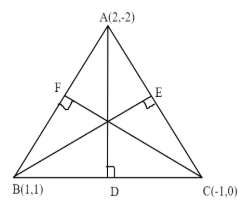Courses
Courses for Kids
Free study material
Free LIVE classes
MoreLIVE
Join Vedantu’s FREE Mastercalss

# Find the equations to the altitudes of the triangle whose angular points are $A\left( {2, - 2} \right),B\left( {1{\text{ }},1} \right),C\left( { - 1,0} \right)$ .Verified
360.3k+ views
Hint: In order to solve these type of question, we have to simply find out the slopes $m$ between two points i.e. $AB,AC,BC$ using formula $m = \dfrac{{{y_2} - {y_1}}}{{{x_2} - {x_1}}}$ and then convert them into slopes of the altitudes $AD,BE,CF$ by using ${m_{AD}} = - \dfrac{1}{{{m_{BC}}}}$ , ${m_{BE}} = - \dfrac{1}{{{m_{AC}}}}$ , ${m_{CF}} = - \dfrac{1}{{{m_{AB}}}}$ after that substitute the value of ${m_{AD}},{m_{BE}},{m_{CF}}$ in equation for altitude between two points $y - {y_1} = m\left( {x - {x_1}} \right)$ .Given points are,
$A\left( {2, - 2} \right),B\left( {1{\text{ }},1} \right),C\left( { - 1,0} \right)$
Now we can find the slope of $A\left( {2, - 2} \right),B\left( {1,1} \right)$ by using the formula,
$m = \dfrac{{{y_2} - {y_1}}}{{{x_2} - {x_1}}}$
${m_{AB}} = {\text{ }}\dfrac{{1 - \left( { - 2} \right)}}{{1 - 2}}$
Or ${m_{AB}} = - 3$
Therefore, using ${m_{CF}} = - \dfrac{1}{{{m_{AB}}}}$
${m_{CF}} = {\text{ }}\dfrac{1}{3}$
Using $y - {y_1} = m\left( {x - {x_1}} \right)$
$y - 0 = \dfrac{1}{3}\left( {x - \left( { - 1} \right)} \right)$
Or $3y = x + 1$
Or $x - 3y + 1 = 0 - - - - - - \left( 1 \right)$
Similarly Slope of $B\left( {1{\text{ }},1} \right),C\left( { - 1,0} \right)$ is
${m_{BC}} = \dfrac{{0 - 1}}{{ - 1 - 1}}$
${m_{BC}} = \dfrac{1}{2}$
Therefore, using ${m_{AD}} = - \dfrac{1}{{{m_{BC}}}}$
${m_{AD}} = - 2$
Using $y - {y_1} = m\left( {x - {x_1}} \right)$
$y - \left( { - 2} \right) = \left( { - 2} \right)\left( {x - 2} \right)$
Or $y + 2 = - 2x + 4$
Or $y + 2x - 2 = 0 - - - - \left( 2 \right)$
Slope of $A\left( {2, - 2} \right),C\left( { - 1,0} \right)$
${m_{AC}} = \dfrac{{0 - \left( { - 2} \right)}}{{ - 1 - 2}}$
Or ${m_{AC}} = - \dfrac{2}{3}$
Therefore, using ${m_{BE}} = - \dfrac{1}{{{m_{AC}}}}$
${m_{BE}} = \dfrac{3}{2}$
Now, using $y - {y_1} = m\left( {x - {x_1}} \right)$
$y - 1 = \dfrac{3}{2}\left( {x - 1} \right)$
Or $\left( {y - 1} \right)2 = 3x - 3$
Or $2y - 2 - 3x - 3 = 0$
Or $2y - 3x + 1 = 0 - - - - - \left( 3 \right)$
Therefore, $\left( 1 \right),\left( 2 \right),\left( 3 \right)$ are the equations of CF , AD , and BE which are altitudes of the given triangle.
Note: Whenever we face these type of question the key concept is that firstly we have to find out the slopes of $AB,AC,BC$ and the convert them into the slopes of altitudes $AD,BE,CF$ and then put them in the equation of altitudes between two points and we will easily get our desired equations.
Last updated date: 15th Sep 2023
Total views: 360.3k
Views today: 6.60k